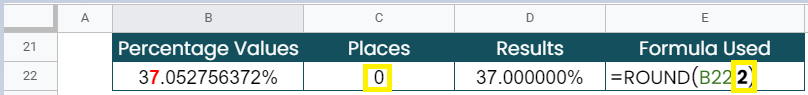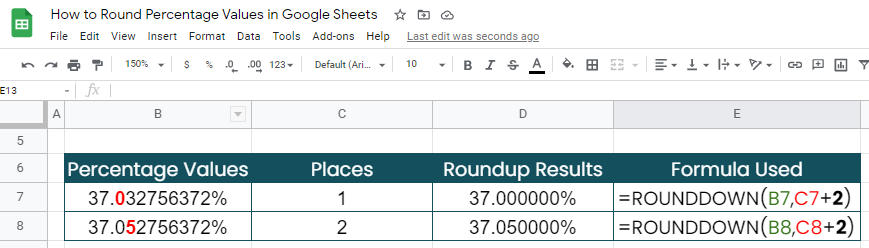# How to Round Percentage Values in Google Sheets

Knowing how to round percentage values in Google Sheets is useful if you want to reduce the decimal places of a percentage value by a specific number.

Meaning, the percentage value is rounded up or rounded down to provide or express a more manageable figure.

There are standard rules related to rounding numbers. Please read our ROUND function, where we explained and provided samples on how to round a value. In this article, we will be concentrating on how to round a percentage value to a certain number of decimal places in Google Sheets.

The rule for rounding percentage values in Google Sheets is as follows:

• The same ROUND function will be used for rounding percentage values
• To round percentage values, add 2 to ‘places’, or the second argument in the ROUND function

Let’s take an example.

Jason, a professor in senior high, needs to report the result of the recently concluded major exam of his students. His data is shown below:His supervisor reminded him that it is standard to a report document that all percentage values are in the nearest hundredths.

Jason applied the ROUND function to be able to come up with the final report document below:This way, the rounded numbers are easier to understand and the calculations become easier to work with, compared to the initial report document.

Watch out for a more advanced tutorial and examples on how you can round percentage values in the coming weeks. Be sure to subscribe to be notified.

Awesome! Let’s begin getting to know more about the process and steps in rounding percentage values in Google Sheets.

## The Anatomy of the ROUND Function used for percentage values

The syntax (the way we write) of the ROUND function for percentage values is as follows:

`=ROUND(percentage value, places + 2)`

Let’s dissect this thing and understand what each of these terms means:

• = the equal sign is just how we start any function in Google Sheets. It is how Google Sheets understand that we are asking it to either do a computation or use a function.
• ROUND() this is our ROUND function. ROUND will take the value as well as the optional places (number of places) to round up the value.
• percentage value is the value that you want to round. Always has the percent sign ‘%’ after the value.
• places + 2 is the number of decimal places to which you wish to round. I’ll explain below why we need to add 2 to the number of places you want to round.

## A Real Example of Rounding Percentage Values

Take a look at the example below to see how ROUND functions are used in Google Sheets for converting percentage values.As you can see, the ROUND function rounds up the percentage value 37.0527564% using different places (number of places).

Please take note that, to round a percentage value, we must keep in mind it’s a decimal value. It means that, for our example percentage, 37.0527564% is equivalent to the decimal value 0.370527564.So to round to the nearest whole percentage, use the ROUND Function with places = 2.This means that, for us to convert a percentage value, we need to let the Google sheet know that we’re trying to convert a percentage value by providing the second argument, places, and adding 2 to it.

Not providing a number for places will round the value using 0 as the default places value. This is the same as providing places a value of -2.In short, please see the table below:This explains the syntax : =ROUND(percentage value, places + 2)

You may make a copy of the spreadsheet using the link I have attached below.

What’s amazing about the method above is that you can even apply the same logic to ROUNDUP and ROUNDDOWN functions.## How to Round Percentage Values in Google Sheets

1. Simply click on any cell to make it the active cell. For this guide, I will be selecting D3, where I want to show my result.1. Next, type the equal sign ‘=‘ to begin the function and then followed by the name of the function, which is our ‘round‘ (or ‘ROUND‘, not case sensitive). For the purposes of this guide, we’ll be just choosing ROUND for now, but the steps shown here work exactly the same for both ROUNDUP and ROUNDDOWN (if you’re interested).

1. Type open parenthesis ‘(‘ or simply hit Tab key to let you use that function.1. Now the fun part! Let’s give our function the first argument, which is the percentage value. You may pass a constant data by typing the exact percentage value after the parenthesis. (Don’t forget to include the ‘%’ to let the Google Sheet know that we’re passing a percentage value)1. To let the Google sheet know that we’re done typing our first argument, we should now type in the delimiter or the character that separates each argument on a function. In this case, type comma,’ followed by the second argument, which is the places.1. Again, since we are trying to convert a percentage value, we need to add 2 to the places we will provide as a second argument. We can type 1 + 2, or simply put 3.

1. Finally, just hit your Enter or Tab key. The cell D3 is now showing you the result or the return value of the ROUND function.1. Notice that, in our example, we provided the exact value, constant, as the arguments of our ROUND function. Alternatively, these constants can be variable or simply cell addresses of your data.

1. In cell D4, follow steps 1-7. Only this time, instead of the actual given percentage value and places, we will use the cell addresses where your percentage value and places are located. In this case, cell B4 and cell C4. Again, don’t forget to add 2 to C4. You may type in C4 + 2 as the second argument.1. Copy the formula down to the remaining rows.That’s pretty much it.  You can now round percentage values in Google Sheets together with the other numerous Google Sheets formulas to create even more powerful formulas that can make your life much easier.Our goal this year is to create lots of rich, bite-sized tutorials for Google Sheets users like you. If you liked this one, you'll love what we are working on! Readers receive ✨ early access ✨ to new content.

##### You May Also Like## How to ROUND Numbers in Google Sheets

The ROUND function in Google Sheets is useful if you want to reduce a value by a specific…## How to Use Spell Check in Google Sheets to Find Misspelled Words

In this article, we’ll learn how to use spell check in Google Sheets to find misspelled words. We’ll…## How To Use CORREL Function in Google Sheets

The CORREL function in Google Sheets is helpful when you need to compute the Pearson product-moment correlation coefficient…## How to Use MAX Function in Google Sheets

The MAX function in Google Sheets is useful when you need to get the maximum value in a…